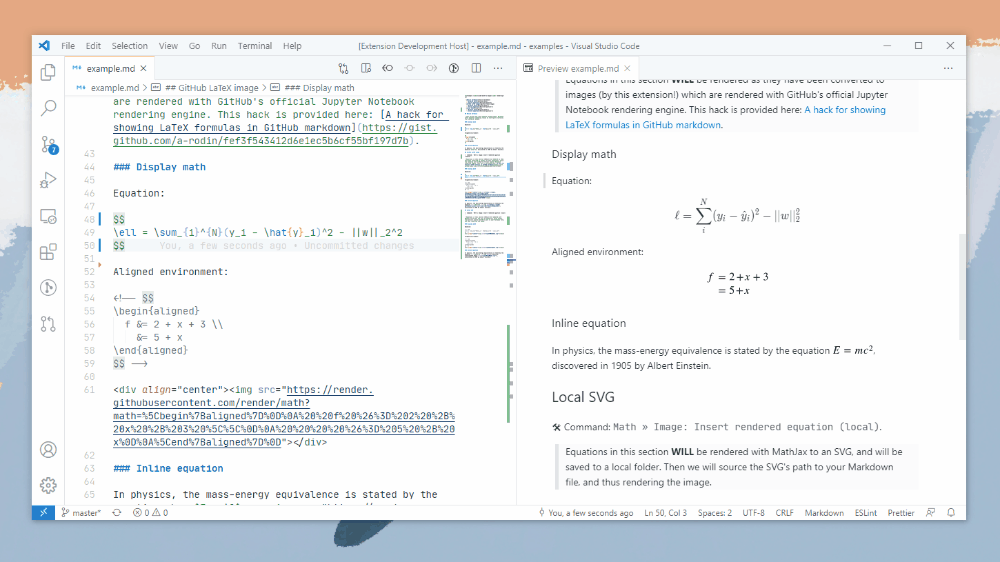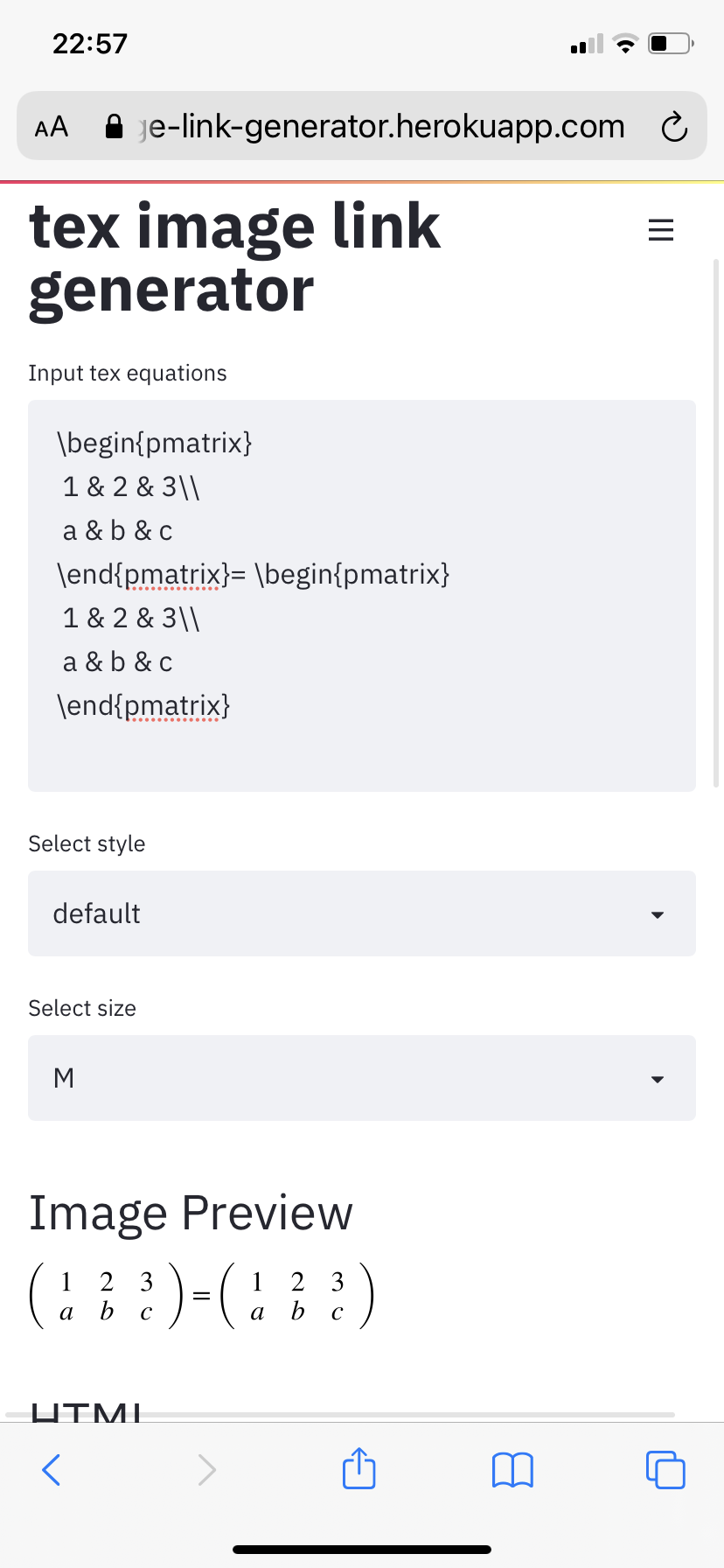{{ message }}

Instantly share code, notes, and snippets.

# a-rodin/A hack for showing LaTeX formulas in GitHub markdown.md

Last active Dec 4, 2020

## Problem

A lot of GitHub projects need to have pretty math formulas in READMEs, wikis or other markdown pages. The desired approach would be to just write inline LaTeX-style formulas like this:

$e^{i \pi} = -1$

Unfortunately, GitHub does not support inline formulas. The issue is tracked here.

## Investigation

However, it does support them in Jupyter notebooks, scroll below to see an example. One might question how does it work in notebooks. It turns out that instead of relying on MathJax as nbviewer does, GitHub's notebooks renderer converts inline math to images with source URLs that look like this:

https://render.githubusercontent.com/render/math?math=e%5E%7Bi%20%5Cpi%7D%20%3D%20-1&mode=inline

Inspecting the URL, it is possible to notice that:

1. The &mode=inline part is not required, the URL still returns the same image without it:

2. Modern browsers encode URLs automatically, thus this link would work as well:

## Solution

So the solution would be to insert this code in Markdown files:

<img src="https://render.githubusercontent.com/render/math?math=e^{i \pi} = -1">

and such an image would be rendered as.

This is more clumsy than just $e^{i \pi} = -1$, but it is still possible to write the formula by hand directly to the Markdown file this way.

Note that this syntax for image insertion would not work because the URL contains spaces:

![formula](https://render.githubusercontent.com/render/math?math=e^{i \pi} = -1)

I made a small app that allows to generate Markdown code from LaTeX using the method described above automatically.

 { "cells": [ { "cell_type": "markdown", "metadata": {}, "source": [ "$e^{i \\pi} = -1$" ] } ], "metadata": { "kernelspec": { "display_name": "Python 3", "language": "python", "name": "python3" }, "language_info": { "codemirror_mode": { "name": "ipython", "version": 3 }, "file_extension": ".py", "mimetype": "text/x-python", "name": "python", "nbconvert_exporter": "python", "pygments_lexer": "ipython3", "version": "3.7.0" }, "toc": { "base_numbering": 1, "nav_menu": {}, "number_sections": false, "sideBar": true, "skip_h1_title": false, "title_cell": "Contents", "title_sidebar": "Contents", "toc_cell": false, "toc_position": {}, "toc_section_display": true, "toc_window_display": false } }, "nbformat": 4, "nbformat_minor": 2 }

###tpenguinltg commented May 10, 2019 • edited

 The Markdown image syntax should work if you replace the spaces with %20. Testing below:###daos20x15 commented Aug 27, 2019

 I wanted to write a formula with a "+" sign, but its apparently not working, any thoughts on how to make the "+" show? 

###a-rodin commented Aug 27, 2019 • edited

 @daos20x15 + symbol is used to escape spaces in URI encoding and the formula has to be either properly escaped or at least don't conflict with possible escaped version created by the browser. The browser replaces spaces by + or %20 before sending to the server, so when it encounters + it assumes that it is already escaped and sends + sign as is, which is decoded by the server as a space. So + should be replaced by encoded version, which is %2B. To be short: a formula with pluses should be written like this   which would be displayed as. To encode other symbols or entire formulas you can use encodeURIComponent function from you browser's developer console.

###daos20x15 commented Aug 27, 2019

 @daos20x15 + symbol is used to escape spaces in URI encoding and the formula has to be either properly escaped or at least don't conflict with possible escaped version created by the browser. The browser replaces spaces by + or %20 before sending to the server, so when it encounters + it assumes that it is already escaped and sends + sign as is, which is decoded by the server as a space. So + should be replaced by encoded version, which is %2B. To be short: a formula with pluses should be written like this   which would be displayed as ![](https://render.githubusercontent.com/render/math?math=e^{i %2B\pi} =x%2B1). To encode other symbols or entire formulas you can use encodeURIComponent function from you browser's developer console. This is very useful. Thanks a lot @a-rodin

###pedrolemoz commented Oct 29, 2019 • edited

 How to use this method to render brackets and sets (from mathematic)? I tryed these: ![formula](https://render.githubusercontent.com/render/math?math=A%20-%20B%20=%20\{x%20\in%20\U%20\mid%20x%20\in%20A%20\land%20x%20\notin%20B%20\}) ![formula](https://render.githubusercontent.com/render/math?math=A,%20B%20\in%20\U,%20x%20\in%20\Z) And I get:But, if I try to add a bracket... ![formula](https://render.githubusercontent.com/render/math?math=\left%20\{%20\right%20\})And if I try to add a set notation... ![formula](https://render.githubusercontent.com/render/math?math=\N\Z\R\Q\C)These aren't sets! How can I get what I want?

###a-rodin commented Oct 30, 2019 • edited

 @pedrolemoz Braces ({}) need to be escaped by \ in LaTeX, square brackets ([]) can be used as is in LaTeX, but they collide with Markdown syntax and thus need to be URI-encoded. I made a small app that converts LaTeX to Markdown code automatically. Here is an example that uses both braces, square brackets, and sets.

###jaimet commented Nov 21, 2019

 Hi. Would you consider making a repo out of this? (I would like to add some content such as corrections and additional documentation, but I don't want to do it via comments on a gist.) Thank you for the original "hack" - it's brilliant!

###chewxy commented Jan 17, 2020

 This is brilliant. Thanks

###Mekahou commented Feb 17, 2020

 This is awesome. Thanks a lot!

###behrouz-bakhtiari commented Feb 24, 2020

 Thank you very much. This is very useful. I am a little disappointed at Github, but this method is a life saver.

###joyjeni commented Mar 20, 2020

 %2B Thankyou It worked

###kartynnik commented Apr 4, 2020

 @a-rodin Why are you using KaTeX for previews in your app? It seems natural to give the "WYSIWYG" feedback by showing the exact image as rendered by the GitHub's renderer.

###manueltonneau commented Apr 5, 2020

 Thank you very much, very cool stuff! If anybody was wondering how to render the equation in a bigger size, just write height="50" width="500" (just adapt the values to your case) after the last " and before >.

###a-rodin commented Apr 5, 2020

 @kartynnik The preview images are returned by GitHub, so they look exactly the same as they would on GitHub. However, in case of parsing errors GitHub doesn't provide an error message, but just returns HTTP 406 error code. In such cases the formulas are additionally parsed using KaTeX and if KaTeX parser detects any error, such an error is displayed to the user to make it easier to fix it. That's why the previews are not instant, but take some time to show up.

###kartynnik commented Apr 5, 2020

 @a-rodin Makes perfect sense, thanks!

###gopal151295 commented May 2, 2020

 @a-rodin This is really great

###ninjakx commented May 11, 2020 • edited

 It's not working for me in .rst file Works here:There It's excluding the circular bracket.

###vrn25 commented May 23, 2020

 @a-rodin is there any way for using $$e^{i \pi} = -1$$?

###psambit9791 commented Jun 1, 2020

 Much appreciation for sharing this. Saved me a good bit of time.

###ghost commented Jun 13, 2020

 This is wonderful. Thank you for posting this!!

###jk13o3lll commented Jun 23, 2020 • edited

 Here is a simple script to convert the equation into html: https://jsfiddle.net/8ndx694g/ For the following example, it works well. \Huge \begin{align*} x&=1\\ x+y&=2\\ P&=\begin{bmatrix}p_1\\p_2\end{bmatrix} \end{align*} 

###psireal9 commented Jun 24, 2020 • edited

 The ![formula][url] doesnt work in an evironment ... (for example if you want to the your equation inside or "")

###mubaraqwahab commented Jul 8, 2020

 Thank you!

###zuyazii commented Jul 30, 2020

 Here is a simple script to convert the equation into html: https://jsfiddle.net/8ndx694g/ For the following example, it works well. \Huge \begin{align*} x&=1\\ x+y&=2\\ P&=\begin{bmatrix}p_1\\p_2\end{bmatrix} \end{align*}  Thank you! It helped me a lot!

###spencerwooo commented Aug 2, 2020 • edited

 Hi guys, me and my friends made a VS Code extension to convert math equations into rendered SVGs here: GitHub- vscode-math-to-image / VS Marketplace - Math to Image. We used the method mentioned in this gist to render external SVGs with GitHub math rendering servers. We also added a feature to render them into local SVGs with MathJax. Feel free to try it out! ❤###masakiaota commented Aug 10, 2020

 https://gist.github.com/a-rodin/fef3f543412d6e1ec5b6cf55bf197d7b#addition-from-2019-10-30 I made a small app that allows to generate Markdown code from LaTeX using the method described above automatically. This link doesn't work... so I made new one https://tex-image-link-generator.herokuapp.com/

###juangburgos commented Aug 27, 2020

 Thank you @masakiaota, you are the best. Unbelievable that github does not prove a built in solution for this. Gatlab has it for years now, although they broke it recently which also sucks.

# Thanks

###hypercube-software commented Sep 23, 2020 • edited

 You may also take a look on my tool: latexMarkdown2Markdown

###Spir4L commented Sep 24, 2020

 @pedrolemoz Braces ({}) need to be escaped by \ in LaTeX, square brackets ([]) can be used as is in LaTeX, but they collide with Markdown syntax and thus need to be URI-encoded. I made a small app that converts LaTeX to Markdown code automatically. Here is an example that uses both braces, square brackets, and sets. Hey, the link is broken!

###Spir4L commented Sep 24, 2020

 https://gist.github.com/a-rodin/fef3f543412d6e1ec5b6cf55bf197d7b#addition-from-2019-10-30 I made a small app that allows to generate Markdown code from LaTeX using the method described above automatically. This link doesn't work... so I made new one https://tex-image-link-generator.herokuapp.com/ It doesn't work for matrices

###masakiaota commented Sep 24, 2020

 https://gist.github.com/a-rodin/fef3f543412d6e1ec5b6cf55bf197d7b#addition-from-2019-10-30 I made a small app that allows to generate Markdown code from LaTeX using the method described above automatically. This link doesn't work... so I made new one https://tex-image-link-generator.herokuapp.com/ It doesn't work for matrices work well in my environment...###francescoapg commented Oct 23, 2020

 Hero! I tried to see you app or web page but it seemed to be shuted down :s

# Display Math Formulae via GitHub (GFM) Markdown

The Future Value equation, F=P(1+(i/n)^n in math format, below uses LaTex math formulas. It's displayed in GitHub (GFM) Markdown. Use LaTex's font size tags to display your equation in sizes ranging from tiny to HUGE.

## Future Value

☛ Since the GFM engine encodes + as space, to include a "plus sign", use %2B ( UTF-8 URL Encoding (Percent Encoding)).
☛ It is not necessary to use spaces in your equation. The LaTex math rendering engine ingnores spaces and uses its own proprietary kerning algorithm to generate the appropriate visual spacing.

Math Markup Renderer Rendered Kind
F=P (1+(i/n)^n HTML-CSS F=P(1+(i/n)^n plain text
$F=P(1+\frac{i}{n})^{nt}$ MathML $F=P(1+\frac{i}{n})^{nt}$ MathJax
F=P(1+\frac{i}{n})^{nt} GFMSVG
F=P(1+\frac{i}{n})^{nt} CodeCogsSVG

## Resizing Formulae Images & %20 URL Space Encoding

Modifier Example Rendered ␣=
\tiny \tiny F=P(1+\frac{i}{n})^{nt}%20
\Tiny \Tiny F=P(1+\frac{i}{n})^{nt}%20
normal F=P(1+\frac{i}{n})^{nt}%20
\large \large F=P(1+\frac{i}{n})^{nt}%20
\Larg1 \Large F=P(1+\frac{i}{n})^{nt}%20
\LARGE \LARGE F=P(1+\frac{i}{n})^{nt}%20
\huge \huge F=P(1+\frac{i}{n})^{nt}%20
\Huge \Huge F=P(1+\frac{i}{n})^{nt}%20

## Resizing Formulae Images & + URL Space Encoding

Modifier Example Rendered ␣+
\tiny \tiny F=P(1+\frac{i}{n})^{nt}+
\Tiny \Tiny F=P(1+\frac{i}{n})^{nt}+
normal F=P(1+\frac{i}{n})^{nt}+
normal* F=P(1+\frac{i}{n})^{nt}+
normal F=P(1+\frac{i}{n})^{nt}+
\large \large F=P(1+\frac{i}{n})^{nt}+
\Larg1 \Large F=P(1+\frac{i}{n})^{nt}+
\LARGE \LARGE F=P(1+\frac{i}{n})^{nt}+
\huge \huge F=P(1+\frac{i}{n})^{nt}+
\Huge \Huge F=P(1+\frac{i}{n})^{nt}+

*WRONG: The + (plus sign) is used instead of its %2B encoded version in the url and doesn't appear in the rendered image.
RIGHT: The + (plus sign) is used instead of its %2B encoded version in the url. Note: CodeCogs renders "normal" at a different scale.

☛ To verify that + space encoding is working, hover over the formula's image until your browser displays the link. Alternatively, right-click on any formula image and click Copy Link Address to capture the link and test for yourself.

# GFM url syntax
# https://render.githubusercontent.com/render/math?math={math_equation}
# equation syntax
F=P(1%2B\frac{i}{n})^{nt}            # normal, valid
\large F=P(1%2B\frac{i}{n})^{nt}     # large: before encoding spaces, invalid
%5Clarge%20F=P(1%2B\frac{i}{n})^{nt} # large: after %20 space and %5C backslash/reverse solidus \ encoding, valid
\large+F=P(1%2B\frac{i}{n})^{nt}     # large: after + space encoding, valid
# e.g. https://render.githubusercontent.com/render/math?math=e%5E%7Bi%5Cpi%7D%20%3D%20-1
#
# CodeCogs url syntax
# https://latex.codecogs.com/svg.latex?{math_equation}
# equation syntax
F=P(1+\frac{i}{n})^{nt}            # normal, valid
\large F=P(1+\frac{i}{n})^{nt}     # large: before encoding spaces, invalid
%5Clarge%20=P(1+\frac{i}{n})^{nt}  # large: after %20 space and %5C backslash/reverse solidus \ encoding, valid
# e.g. https://latex.codecogs.com/svg.latex?%5Clarge%20F=P(1+\frac{i}{n})^{nt}